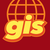# arcpy.GetParameterAsText getting null value if used after arcpy.ExcelToTable_conversion

870
3
09-20-2022 03:13 AMNew Contributor III

Hi everyone,

I recently discovered something strange with arcpy.GetParameterAsText() in a script tool. If the function is used after arcpy.ExcelToTable_conversion, somehow it will not receive any value from the toolbox.

I managed to reproduce this behaviour with the simplest script here.

``````import arcpy,os

if __name__ == '__main__':

param1 = arcpy.GetParameterAsText(0) #gdb
param2 = arcpy.GetParameterAsText(1)

in_excel = r"E:\Python\ghzrzyw_202209\script\new.xlsx"
fgdb = r"D:\Documents\ArcGIS\Default.gdb"
out_table = os.path.join(fgdb, "testtable")
arcpy.env.workspace = fgdb
arcpy.env.OverwriteOutput = True
sheet = "test"
arcpy.ExcelToTable_conversion(in_excel, out_table, sheet)
param3 = arcpy.GetParameterAsText(2)
param4 = arcpy.GetParameterAsText(3)

The messages printed out only contain param 1 and 2.

Param 3 and 4 are NoneType values based on my further test, even though I provided some values as input. This happens regardless of the parameter data types configured with the script tool.if I shift arcpy.ExcelToTable_conversion to after all the arcpy.GetParameterAsText(), then it all works fine. So it would seem that whether arcpy.GetParameterAsText is placed is the main culprit here.

My questions:

1. Is this an expected behaviour? I'm using ArcMap 10.8.1.
2. If so, does it mean that it's always recommended to use arcpy.GetParameterAsText() at the start of the script?

Tags (3)
1 Solution

Accepted SolutionsOccasional Contributor III

My general rule for writing script tools is to structure them like this:

``````def main(param0, param1, param2):
return doSomethingWith(param0) + doOtherThingWith(param1 + param2)

if __name__ == "__main__":
param0 = arcpy.GetParameterAsText(0)
param1 = arcpy.GetParameterAsText(1)
param2 = arcpy.GetParameterAsText(2)
param3 = main(param0, param1, param2)
arcpy.SetParameterAstext(3, param3)``````

This has two big benefits. First, you ensure all the parameters are yanked out of the aether and loaded into proper variables before you run your task. Second, you now have a main function that isn't strictly dependent on parameter state, which means you can write tests for it or call the function from other scripts without going through importing a toolbox.

3 RepliesbyMVP Esteemed Contributor

There is no documentation as to why this would be.... except for the line...

When the tool is executed, the parameter values are sent to your tool's source code. Your tool reads these values and proceeds with its work.

Setting script tool parameters—ArcGIS Pro | Documentation

which suggests to me that they should be grouped together at the beginning of a script so the information can be collected before anything else is done.

... sort of retired...New Contributor III

Thanks Dan. In fact we used to have several other lines in between, including arcpy.CreateFileGDB_management, arcpy.ValidateTableName and others, but none of them had this issue when used before arcpy.GetParameterAsText.

If it's indeed the case that arcpy.GetParameterAsText should only be used at the start of the script, we'll just adhere to this good practice then.

Thanks again!Occasional Contributor III

My general rule for writing script tools is to structure them like this:

``````def main(param0, param1, param2):
return doSomethingWith(param0) + doOtherThingWith(param1 + param2)

if __name__ == "__main__":
param0 = arcpy.GetParameterAsText(0)
param1 = arcpy.GetParameterAsText(1)
param2 = arcpy.GetParameterAsText(2)
param3 = main(param0, param1, param2)
arcpy.SetParameterAstext(3, param3)``````

This has two big benefits. First, you ensure all the parameters are yanked out of the aether and loaded into proper variables before you run your task. Second, you now have a main function that isn't strictly dependent on parameter state, which means you can write tests for it or call the function from other scripts without going through importing a toolbox.